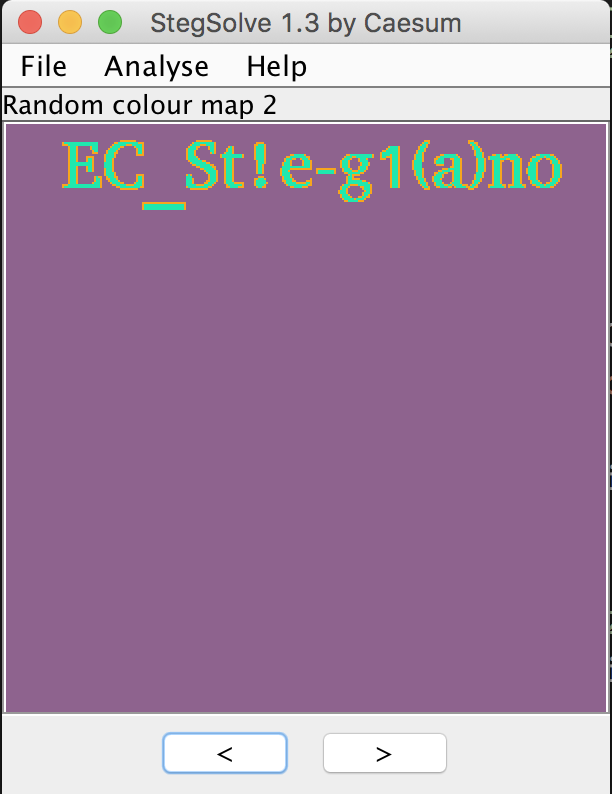# Securinets CTF 2018 Crypto Writeup

I’ve been looking for crypto challenges lately. In this CTF challenge, there are three crypto challenges (with some steganography for the last one).

## Looser - 150 Points

We got flag.png.crypt, I think that this image may be encrypted with xor of some bytes. We know (by searching) that PNG file header starts its 8 bytes with 89 50 4E 47 0D 0A 1A 0A. So we try to find the key.

enc = open('flag.png.crypt').read()
ks = []
for i in range(8):
print ks


The result is

[238, 238, 238, 238, 238, 238, 238, 238]


So we know that the key is just a single byte (with ordinal integer 238). After get the key we can xor the key with every bytes of encrypted image and get the flag.

enc = open('flag.png.crypt').read()
# 1st PNG byte

def xor(a,b):
return ''.join([chr(ord(x)^ord(y)) for x,y in zip(a,b)])

dec = ''
for i in enc:
dec += xor(k, i)

with open('flag.png', 'w') as f:
f.write(dec)
f.close()


And the flag is in the flag.png.## The Worst RSA Joke - 350 Points

I was tried to find a way to factor its modular n, and all techniques I tried were failed. I decide to go back to the description and found that it uses single prime. Which means n is prime and I can’t factor it for sure. Back to the basic of RSA, we know that private key (d) is computed by

$$d \equiv e^{-1} \mod φ(n)$$

and we know that for any prime p, it holds

$$φ(p) = p-1$$

We have e and φ(n)(n-1),so we cat get the private key easily.

from Crypto.PublicKey import RSA
from Crypto.Util.number import long_to_bytes, bytes_to_long
from gmpy import invert
p, e = pubkey.n, pubkey.e

d = invert(e, p-1)
m = pow(c, d, p)
flag = long_to_bytes(m)
print flag


And we get the output which contains our flag.

The empire secret system has been exposed ! The top secret flag is : Flag{S1nGL3_PR1m3_M0duLUs_ATT4cK_TaK3d_D0wn_RSA_T0_A_Sym3tr1c_ALg0r1thm}


Flag: Flag{S1nGL3_PR1m3_M0duLUs_ATT4cK_TaK3d_D0wn_RSA_T0_A_Sym3tr1c_ALg0r1thm}

## Improve the quality - 800 Points

From the description, the secret message was splitted into parts (e.g. 123456 => 12, 34, 56). And each part is the secret key for each given point on the Elliptic Curve.

$$Q1 = k1 * G \newline Q2 = k2 * G \newline …$$

We have to combine k1, k2, … to get the secret message.

### 1. Find B

With A, p, and (x,y) coordinate of any point on the curve, we can find B with

$$y^2 \equiv x^3 + Ax + B\mod p\newline B \equiv y^2 - x^3 - Ax\mod p$$

I choose (x,y) from a generator G, compute above equation. We will get B as 618.

### 2. Find each secret key

Since it is just a small number, we can compute Discrete Logarithm directly via discrete_log in Sagemath.

#!/usr/bin/env sage
A = 658974
p = 962280654317
B = Mod(339109212996**2 - 518459267012**3 - A*518459267012 , p)
E = EllipticCurve(GF(p), [A, B])
G = E(518459267012, 339109212996)

Qs = [(656055339629, 670956206845),
(714432985374, 30697818482),
(519532969453, 833497145865),
(606806384185, 353033449641),
(370553209582, 211121736115),
(95617246846, 666814491609),
(474872055371, 795112698430),
(249845085299, 222352033875),
(850954431245, 810446463695),
(188731559428, 877002121896),
(168665615402, 464872506873),
(26722558561, 269217869309),
(16403346294, 478534963882),
(539749282946, 332444159141),
(932295517649, 23439478940),
(765194933041, 920187938377),
(853124087439, 845601917928),
(246454416048, 212483699689),
(312547608490, 688107262695),
(43261158649, 439444472742),
(320785434805, 477080449838),
(741706320740, 672809544395),
(361762297756, 858805805323),
(782235980044, 600673464737),
(69196762074, 327427680437),
(876001563166, 573218279075),
(117946101727, 954797129239),
(771781111553, 314018907599),
(579549799021, 322325160055),
(857081196493, 464260539273),
(852938568103, 429083796488),
(850954431245, 810446463695),
(55203632714, 255470537391),
(600464434215, 605840305721),
(620532163623, 575613893944),
(215810002861, 481354983411),
(538481263994, 666638294130),
(528666082457, 895034116069),
(296218553972, 899557390183),
(428618251485, 445768511836),
(632412058600, 685699421425),
(634041855232, 495546745721),
(570481762204, 252944477333),
(760959783781, 435626456209)]

Ks = []

for q in Qs:
Q = E(q)
Ks += [discrete_log(Q, G, operation='+')]


### 3. Find the (not really) fla

The hint said that after we get the key, split it to 2 chars (123456 => [12, 34, 56]) so I think that each element in the list can be transform with chr, and it is.

K = ''.join([str(i) for i in Ks])

flag = ''
for i in range(0,len(K),2):
flag += chr(int(K[i:i+2]))

print flag


The output is

CONVERT THIS TO LOWER CASE FIRST :
THIS IMAGE CONTAINS THE FLAG, TRY TO GET IT
THE SUBMITTED FLAG MUST BE IN THIS FORMAT:
FLAG-EC[WHAT YOU'LL FIND IN THE IMAGE]
IMAGE URL:
HTTP://CRYPTO.CTFSECURINETS.COM/1/STEG-PART.PNG


Here is not the end. We need some more work to get the flag.

### 4. Play with Steg

After converting to the lower case and following the link, we get this image.When it comes to steganography, I usually go to StegSolve as my first choice. And it doesn’t disappoints me.Flag: flag-ec[EC_St!e-g1(a)no]